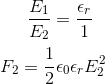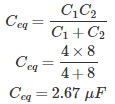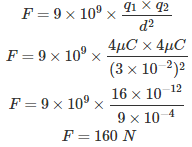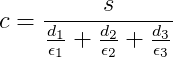# MCQs on Electric Circuits

##### Page 21 of 63. Go to page 1 2 3 4 5 6 7 8 9 10 11 12 13 14 15 16 17 18 19 20 21 22 23 24 25 26 27 28 29 30 31 32 33 34 35 36 37 38 39 40 41 42 43 44 45 46 47 48 49 50 51 52 53 54 55 56 57 58 59 60 61 62 63
01․ A parallel plate capacitor with air as dielectric, force between plate is F. If capacitor is now immersed water of dielectric εr, force between plate will be –
εr/F.
F.
0.
F εr.02․ If c1 = 8 μF, c2 = 4 μF, c3 = 7 μF c1, c2 are in series with c3 in parallel; effective capacitance will be
2.21 μF.
3 μF.
9.67 μF.
6 μF.

When C1 and C2 are in series, then,This is parallel to the C3, So then the equivalent capacitance will be Ceq + C3.

03․ A capacitor having a mica dielectric εR = 6, a plate area of a 10 inches2 and a separation 0.01 inch. Find the value of C
1.349 μF.
1.349 nF.
1.349 F.
0 F.

s = 10 × (0.0254)2 d = 0.1 × (0.0254) c = εR s/d.

04․ Two point charges of 4μC are placed at a distance of 3 cm apart from each other in free space. Force acting on two charges will be
100 N
160 N
400 N
0.02 N

From Coulomb's Law,05․ A parallel plate capacitor contain there dielectric layers of 1, 2 and 3 respectively and thickness of dielectric layers are 0.2 mm, 0.3 mm and 0.4 mm respectively. If area of plate is 20 cm2. What is the value of capacitance?
30 pF.
32 pF.
36.6 pF.
None of them.06․ Under resonance condition the power factor of a system is
unity
lagging
any of the above

The elements present in electrical systems are resistor, inductor and capacitor. Inductor stores the energy in the form of magnetic field and capacitor stores the energy in the form of electric field. When these two energies are equal in a system, large oscillations will occur and this is called resonance. Consider a simple resistor, inductor and capacitor series circuit. Total voltage V = Vr + j(Vl-Vc) Where, Vr = Voltage across resistor Vl = Voltage across Inductor Vc = Voltage across capacitor Resonance will occur when Vl = Vc. Therefore the system voltage is only Vr and current is in phase with voltage across resistor. Therefore, under resonance condition the power factor of a system is unity.

07․ Reactive power drawn by a pure resistor is
0
minimum
maximum
none of the above

Reactive power Q = VI sinsφ Where, V = voltage cross resistor I = current through resistor φ = phase angle between voltage and current When a pure resistor is connected across a voltage source, current is in phase with the voltage. Therefore, Q =VI sin0o Q = 0.

08․ The time required for a pulse to change from 10 % to 90 % of its maximum value is known as
decay time.
rise time.
run time.
fall time.

Rise time is the time required for a over damped system to move from 10% to 90% of its peak value, it is also known as maximum peak overshoot.

09․ Fall time is the time required for a pulse to decrease from 90 to ________% if its maximum values
5
10
15
20

For an under-damped system a 90% to 10% decay is known as the fall time or decay time.

10․ An electrical motor has rating of 10 MVA at 0.8 lag, represented as series RL circuit at a 50 MVA base. Find the impedance value?
(2 + j4) pu
(4 + j3) pu
(3 + j4) pu
(4 + j2 ) pu

When load MVA and power factor are given: If load is represented with equivalent RL circuit, Zpu = Rpu + jXpu = (Sb/S(cosφ + j sinφ))* Where, Zpu = Per unit impedance Rpu = Per unit resistance Xpu = Per unit reactance Sb = Base impedance S = Motor rating cosφ = Power factor Zpu = (50/10(0.8 + j0.6)* = (4 + j3) pu.

<<<1920212223>>>